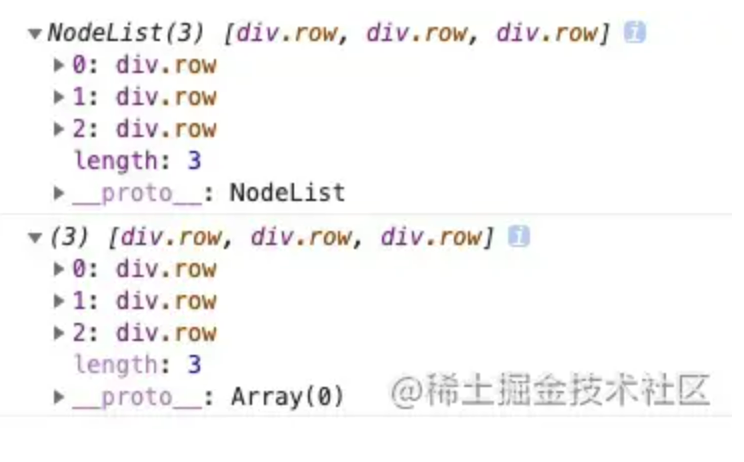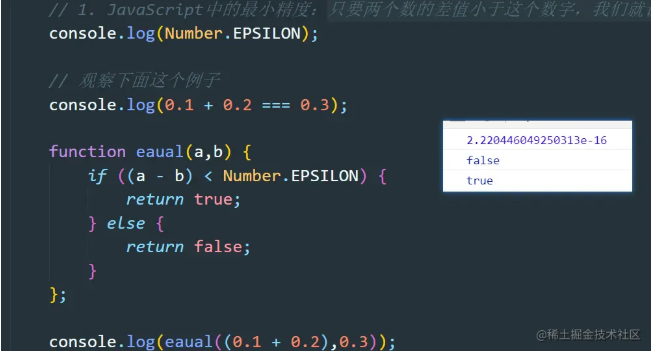# ES6入门之数值的扩展

+关注继续查看

### 1. 二进制和八进制表示法

ES6提供了二进制和八进制数值的新写法，分别用前缀 0b(0B) 和 0o(0O)表示 如下：

0b111110111 === 503 // true
0o767 === 503 // true

### 2. Number.isFinite(), Number.isNaN()

ES6在number上提供了 Number.isFinite(), Number.isNaN()两个方法，前者用来检查数值是否为有限的，后者用来检查一个值是否为NAN，如下：

Number.isFinite(15); // true
Number.isFinite(0.8); // true
Number.isFinite(NaN); // false
Number.isFinite(Infinity); // false
Number.isFinite(-Infinity); // false
Number.isFinite('foo'); // false
Number.isFinite('15'); // false
Number.isFinite(true); // false

Number.isNaN(NaN) // true
Number.isNaN(15) // false
Number.isNaN('15') // false
Number.isNaN(true) // false
Number.isNaN(9/NaN) // true
Number.isNaN('true' / 0) // true
Number.isNaN('true' / 'true') // true

### 3. Number.parseInt(), Number.parseFloat()

ES6 将全局方法parseInt()和parseFloat()，移植到Number对象上面，行为完全保持不变。减少全局性方法，使得语言逐渐模块化。如下：

// ES5的写法
parseInt('12.34') // 12
parseFloat('123.45#') // 123.45
// ES6的写法
Number.parseInt('12.34') // 12
Number.parseFloat('123.45#') // 123.45

#### 4. Number.isInteger()

Number.isInteger()用来判断一个数值是否为整数，如下：

Number.isInteger(25) // true
Number.isInteger(25.1) // false
Number.isInteger(25.0) // true 整数和浮点数采用相同的存储方式

### 5. Number.EPSILON

Number.EPSILON 用来表示1 与 大于1的最小浮点数之间的差，实际上就是JavaScript能够表示的最小精度。

function withinErrorMargin (left, right) {
return Math.abs(left - right) < Number.EPSILON * Math.pow(2, 2);
}
0.1 + 0.2 === 0.3 // false
withinErrorMargin(0.1 + 0.2, 0.3) // true
1.1 + 1.3 === 2.4 // false
withinErrorMargin(1.1 + 1.3, 2.4) // true

### 6. 安全整数和 Number.isSafeInteger()

JavaScript能够精准表示的整数范围在 -2^53到2^53之间（不含两个端点），ES6引入了Number.MAX_SAFE_INTEGER和Number.MIN_SAFE_INTEGER这两个常量，用来表示这个范围的上下限。而Number.isSafeInteger() 就是判断一个整数是否在这个范围之内。

### 7. Math 对象的扩展

ES6 在 Math 对象上新增了 17 个与数学相关的方法。所有这些方法都是静态方法，只能在 Math 对象上调用。

###### Math.trunc()

Math.trunc(4.1) // 4
Math.trunc(4.9) // 4
Math.trunc(-4.1) // -4
Math.trunc(-4.9) // -4
Math.trunc(-0.1234) // -0
// 非数值
Math.trunc('123.456') // 123
Math.trunc(true) //1
Math.trunc(false) // 0
Math.trunc(null) // 0
// 空值和无法截取的值
Math.trunc(NaN);      // NaN
Math.trunc('foo');    // NaN
Math.trunc();         // NaN
Math.trunc(undefined) // NaN
###### Math.sign()

// 返回五种结果：
-     参数为正数，返回+1；
-     参数为负数，返回-1；
-     参数为 0，返回0；
-     参数为-0，返回-0;
-     其他值，返回NaN。
Math.sign(-5) // -1
Math.sign(5) // +1
Math.sign(0) // +0
Math.sign(-0) // -0
Math.sign(NaN) // NaN
Math.sign('')  // 0
Math.sign(true)  // +1
Math.sign(false)  // 0
Math.sign(null)  // 0
Math.sign('9')  // +1
Math.sign('foo')  // NaN
Math.sign()  // NaN
Math.sign(undefined)  // NaN
###### Math.cbrt()

Math.cbrt(-1) // -1
Math.cbrt(0)  // 0
Math.cbrt(1)  // 1
Math.cbrt(2)  // 1.2599210498948734

### 8. 对数方法

###### 8.1 Math.expm1()

Math.expm1(x)返回 ex - 1，即Math.exp(x) - 1。

###### 8.2 Math.log1p()

Math.log1p(x)方法返回1 + x的自然对数，即Math.log(1 + x)。如果x小于-1，返回NaN。

###### 8.3 Math.log10()

Math.log10(x)返回以 10 为底的x的对数。如果x小于 0，则返回 NaN。

###### 8.4 Math.log2()

Math.log2(x)返回以 2 为底的x的对数。如果x小于 0，则返回 NaN。

### 9. 双曲函数方法

ES6 新增了 6 个双曲函数方法。

Math.sinh(x) 返回x的双曲正弦（hyperbolic sine）
Math.cosh(x) 返回x的双曲余弦（hyperbolic cosine）
Math.tanh(x) 返回x的双曲正切（hyperbolic tangent）
Math.asinh(x) 返回x的反双曲正弦（inverse hyperbolic sine）
Math.acosh(x) 返回x的反双曲余弦（inverse hyperbolic cosine）
Math.atanh(x) 返回x的反双曲正切（inverse hyperbolic tangent）

### 10. 指数运算

ES6 新增了指数运算符（**），而且是从右往左计算。

2 ** 3  // 16
2 ** 2 ** 3  === 2 ** (2 ** 3)  //  256
let a = 1.5  a** = 2  ===  a = a*a
let b =4     b** = 3  ===  b = b* b* bES6入门之数组的扩展
ES6入门之数组的扩展
0 0ES6入门之字符串的扩展
ES6入门之字符串的扩展
0 0es6字符串扩展
es6字符串扩展
0 0ES6入门之函数的扩展
ES6入门之函数的扩展
0 0ES6 新特性 扩展运算符(...)详解
ES6 新特性 扩展运算符(...)详解
0 0ES6中扩展运算符的8种用法

0 0ES6—47：ES6中的数值拓展
ES6—47：ES6中的数值拓展
0 0ES6 对数组的扩展

934 0

《Pulsar 2.8.0 功能特性概述及规划》